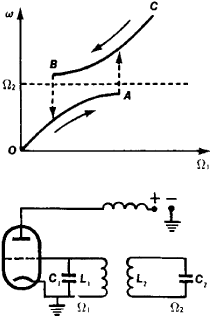Frequency Pulling

frequency pulling

[′frē·kwən·sē ‚pu̇l·iŋ]
(electronics)
A change in the frequency of an oscillator due to a change in load impedance.

Frequency Pulling

the dependence of the trequency of self-oscillations in a two-resonator oscillatory system (Figure 1) on the direction of the change in the tuning of one of the resonators. As Ω1 increases, the oscillator frequency ω increases from O toward A, and when Ω1 decreases, ω decreases from C toward B. At the points A and B an abrupt change in the frequency occurs (the dotted arrows).Figure 1. Change in the frequency ω of a double-circuit electrical oscillator as the frequency ω,1 of the circuit C1L1, changes. The frequency ω,2 of the circuit C2L2 is constant

Frequency pulling takes place when the frequencies Ω1 and (Ω2 are close together. It is used in technology to stabilize the frequency of single-resonator oscillators (such as a klystron). In this case the oscillator is connected to a supplementary resonator with a high quality factor. Owing to the frequency pulling the oscillator frequency ω is maintained close to the frequency of the supplementary resonator Ω2. [9-1151-4; updated]

References in periodicals archive ?
The frequency pulling and the external quality factor were found to be 900 and 1930 kHz, respectively, whereas the frequency pushing for a maximum of 1 V was 125 kHz, that is approximately 0.
A graphical representation of the frequency pulling in a magnetron, along with frequency pushing, can be obtained from a Rieke diagram.
Stability is achieved using a high Q ceramic resonator in a low noise silicon FET circuit with the output buffered to minimize frequency pulling.
Load frequency pulling is reduced by decoupling the load with a low reactance capacitor in parallel with the load.
Frequency pulling is 200 kHz into an SWR of 2 through all phases and frequency pushing is 20 kHz/V.
A high SWR or unstable load not only causes VCO frequency pulling, but will also sometimes produce rapidly accelerated regions of the tuning curve (voltage-to-frequency transfer function).
Frequency pulling is a measure of the frequency change due to a non-ideal load.
When frequency pulling is significant enough to shift the VCO outside of its frequency range, a wide band VCO can more easily accommodate the correction to the frequency shift.
8) Frequency pushing [Hz/V] and frequency pulling [Hz p-p]
Site: Follow: Share:
Open / Close